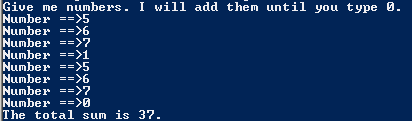# Assignment 67; NumsAndSums

## Code

```    // Donovan Rich
// Period 6
// Adding Values in a Loop
// NumsAndSums.java
// 12/9/2015

import java.util.Scanner;
public class NumsAndSums
{
public static void main ( String[] args )
{
Scanner keyboard = new Scanner(System.in);
int num=1, sum=0;
System.out.println( "Give me numbers. I will add them until you type 0." );
while (num != 0 )
{
System.out.print( "Number ==>" );
num = keyboard.nextInt();
sum += num;
}

System.out.println( "The total sum is " + sum + "." );

}
}
```

### Picture of the output